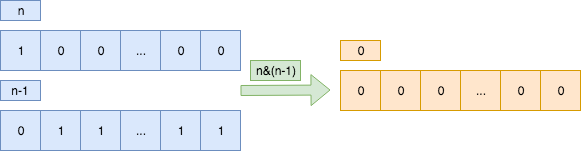# 判断2的幂次数 Power of Two

## 题目

Given an integer, write a function to determine if it is a power of two.

Example 1:

``````Input: 1
Output: true
Explanation: 20 = 1
``````

Example 2:

``````Input: 16
Output: true
Explanation: 24 = 16
``````

Example 3:

``````Input: 218
Output: false
``````

## 思路&题解``````public boolean isPowerOfTwo(int n) {
return n >= 1 && (n&(n-1)) == 0;
}
``````